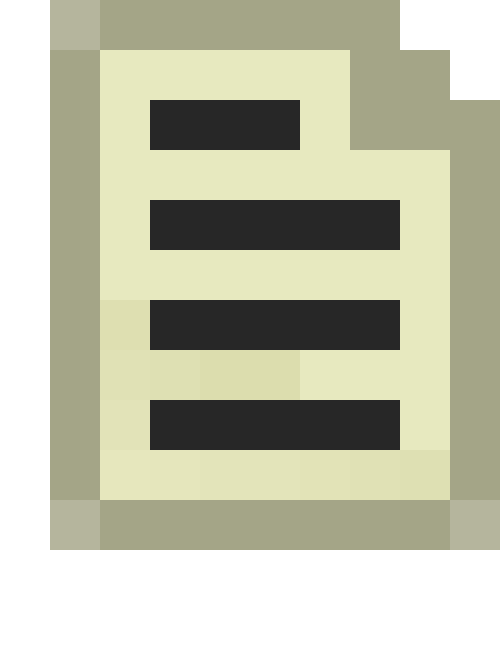Particle System Forces

Particle System Forces apply a force to your particle system.

Pull towards control point

Applies a force around a control point. Internal?

amount of force
Amount of force applied to particles.
falloff power
Determines how long of a range from the control point to stop applying the force.
control point number
Which control point to apply the force around.

random force

Applies a random velocity on all particles.

min force
Minimum force to apply, in XYZ format
max force
Maximum force to apply, in XYZ format

twist around axis

Twists particles around a certain axis

amount of force
Amount of force to apply when a particle is near the axis
twist axis
Location of the axis in XYZ format
object local space axis 0/1
If 1, uses local space axis. If 0, uses world axis?Note:If 0 it seems to work fine...